### Direct Methods: Gauss-Jordan Elimination

### The Method

Another method that is rooted in the Gauss elimination method is the Gauss-Jordan elimination method. Two steps are added to the previous algorithm. The first step is that each pivot row is normalized by the pivot element. The second step is that the coefficients above the pivot element are also eliminated. This results in not needing the backward substitution step. The following example illustrates the method. Consider the system of equations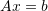defined as: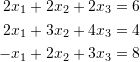The first row is normalized: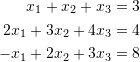The first (pivot) row is used to eliminate the coefficients of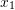in the second and third rows: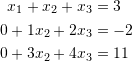The second row is already normalized and can be used to eliminate the coefficients of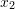in the third equation: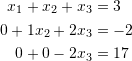It will also be used to eliminate the coefficients ofin the first equation: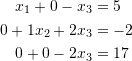The third pivot element will now be normalized: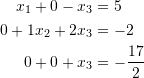The third row is used to eliminate the coefficients of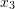in the second and first equations: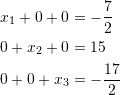The right hand side is the required solution! In matrix form the following are essentially the steps above: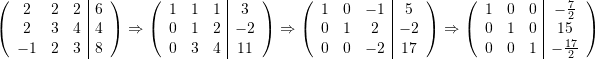The same issue of zero pivot applies to this method and another step of “pivoting” could be added to ensure no zero pivot is found in the matrix.

View Mathematica Code
GJElimination[A_, b_] :=
(n = Length[A];
G = Transpose[Insert[Transpose[A], b, n + 1]];
For[k = 1, k <= n,
k++, (G[[k]] = G[[k]]/G[[k, k]];
Do[G[[i]] = G[[i]] - G[[i, k]]*G[[k]], {i, 1, k - 1}];
Do[G[[i]] = G[[i]] - G[[i, k]]*G[[k]], {i, k + 1, n}])];
x = G[[All, n + 1]])
A = {{1, 2, 3, 5}, {2, 0, 1, 4}, {1, 2, 2, 5}, {4, 3, 2, 2}};
b = {-4, 8, 0, 10};
GJElimination[A, b]

View Python Code
import numpy as np
def GJElimination(A,b):
n = len(A)
G = (np.vstack([A.astype(np.float).T, b.astype(np.float)])).T
for k in range(0,n):
G[k] = G[k]/G[k][k]
for i in range(k):
G[i] = G[i] - G[i][k]*G[k]
for i in range(k+1,n):
G[i] = G[i] - G[i][k]*G[k]
x = G[:,n]
return x
A = np.array([[1, 2, 3, 5], [2, 0, 1, 4], [1, 2, 2, 5], [4, 3, 2, 2]])
b = np.array([-4, 8, 0, 10])
GJElimination(A, b)


The following link provides the MATLAB code for implementing the Gauss-Jordan Elimination Method.